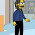## Friday, February 18, 2011

### An early LISP program (circa Feb. 1960)

```       TST M948-371-P. FOX-EXERCISE 1
DEFINE
(((COLLAPSE,(LAMBDA,(L),(COND,
((ATOM,L), (CONS,L,NIL))
((NULL,(CDR,L)),
(COND,((ATOM,(CAR,L)),L),(T,(COLLAPSE,(CAR,L)))))
(T,(APPEND,(COLLAPSE,(CAR,L)),(COLLAPSE,(CDR,L))))
))))) ()
COLLAPSE ((((A,B),((C))),((D,(E,F)),(G),((H))))) ()
COLLAPSE ((A,(B,(C,(D,(E))),F,(G,(H,J))))) ()
COLLAPSE ((((((A),B),C),D),E)) ()
STOP))))))))))STOP
FIN M948-371-P. FOX-EXERCISE 1```
Notes:
1. This example and the quotes below were taken from the LISP I manual by Phyllis Fox. The output from the program contains these two lines:
`APPLY OPERATOR AS OF SEPTEMBER 1, 1959`
`THE TIME IS NOW 2/16 1139.5`
so we can date the sample run.
2. Each line above was punched on a separate card.
3. The `TST` (or `SET`) card indicates the beginning of the program. The `FIN` card indicates the end.
4. This is followed by triplets of the form <function>, <argument-list>, <environment>. Instead of `read`, `eval`, `print`, the main loop is more like `read`, `read`, `read`, `apply`, `print`.
5. The `STOP` card has lots of extra close parens in order to keep the reader from consuming the entire card deck if the programmer didn't correctly close all the parens.
6. — “The read program reads until it finds the correct number of final parenthesis.
7. S-expressions were originally supposed to have commas between the elements. The LISP I manual explains: “The commas in writing S-expressions may be omitted. This is an accident.” The author of the reader took a shortcut and treated whitespace as commas. “The current version of the read program interprets a blank (space) as a comma, so that no blanks are allowed within atomic symbols.
8. LISP II will be able to handle both integers and floating point numbers, but in LISP I integers are not allowed. ... Numbers are brought into a LISP program by being defined within S-expressions, and they must always be quoted. For example, the following expression is correct, `(SUM,(QUOTE,0.6),X)`
9. Values of compiled functions are computed about 60 times faster than the S-expressions for the functions could be interpreted... After a function has been compiled, it can be used as if it had been defined, but of course it will run much faster than it would have as an interpreted expression.

1.Another example is:

http://www.informatimago.com/develop/lisp/small-cl-pgms/wang.html

A driver is defined there to interpret directly the card decks (but the wang program didn't use environment lists, so the driver doesn't take them into account, it would have to be modified to interpret the collapse deck).

2.I would have written it like this:

(define collapse
(lambda (l)
(cond ((pair? l) (append (collapse (car l))
(collapse (cdr l))))
((null? l) '())
(else (list l)))))

3.... and I would have been wrong. This is correct:

(define collapse
(lambda (l)
(cond ((pair? l)
(if (pair? (car l))
(append (collapse (car l))
(collapse (cdr l)))
(cons (car l) (collapse (cdr l)))))
((null? l) '())
(else (list l)))))